# Structure

(diff) ← Older revision | Latest revision (diff) | Newer revision → (diff)

A synonym for algebraic system. Similarly, substructure is a synonym for "subsystem" , and elementary substructure is a synonym for "elementary subsystem" (cf. Model theory). Ifis a first-order language of signatureandis an algebraic system of signature, thenis called an-structure.

A first-order languageis uniquely determined by its signature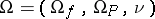(cf. Algebraic system). Therefore, in a more informal way of writing, many authors describeby just giving the setof function symbols (also called "functionals" ) and relation symbols (also called "predicates" ). Here, one omits the mapping, which assigns to every symbol its arity, that is, the number of variables of the function or relation which interprets the symbol in a structure. This is justified when the arity can be read off from the symbol by convention. It is even common to write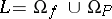. For example,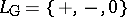is called the language of groups (additively written),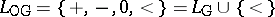is the language of ordered groups,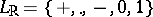is the language of rings with, andis the language of ordered rings. Here, "+" and "" are binary function symbols, "-" is a unary function symbol (for additive inversion), "<" is a binary relation symbol, and "0" and "1" are constant symbols. A constant symbol, belonging to, is a-ary function symbol, but often constant symbols are considered separately. The field axioms can be formulated in the language of rings, but one can also use the language of fields obtained fromby adjoining a unary function symbol for multiplicative inversion.

If the languageis given by a set of relation symbols, function symbols and constant symbols, then an-structureis a set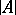, called the universe of the structure, together with relations and functions onand elements of(constants) which interpret the corresponding symbols of. Often,is identified with; for example,may denote the set of real numbers as well as the field of real numbers. In this spirit, an element ofis also called an element of, and the cardinality of a structure is defined to be the cardinality of its universe.

If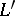is a language containing(as sets of symbols) and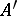is an-structure such thatand the interpretations of the relation, function and constant symbols ofare the same inas in, thenis called an expansion of, andis called a reduct of. For example, a field is an expansion of the underlying additive group, and this group is a reduct of the field.

If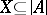, then to add a name for every element into the language, one passes to the expansion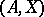of, which has signature(cf. Model theory; Elimination of quantifiers).

How to Cite This Entry:
Structure. Encyclopedia of Mathematics. URL: http://encyclopediaofmath.org/index.php?title=Structure&oldid=14175
This article was adapted from an original article by F.-V. Kuhlmann (originator), which appeared in Encyclopedia of Mathematics - ISBN 1402006098. See original article